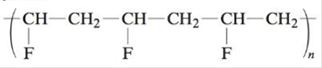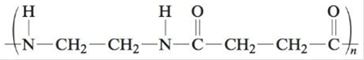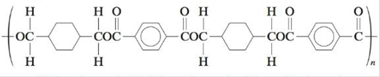# What monomer(s) must be used to produce the following polymers? a. b. c. d. e. f. (This polymer is Kodel, used to make fibers of stain-resistant carpeting.) Classify these polymers as condensation or addition polymers. Which are copolymers?### Chemistry: An Atoms First Approach

2nd Edition
Steven S. Zumdahl + 1 other
Publisher: Cengage Learning
ISBN: 9781305079243

#### Solutions

Chapter
Section### Chemistry: An Atoms First Approach

2nd Edition
Steven S. Zumdahl + 1 other
Publisher: Cengage Learning
ISBN: 9781305079243
Chapter 21, Problem 72E
Textbook Problem
103 views

## What monomer(s) must be used to produce the following polymers?a.b.c.d.e.f.(This polymer is Kodel, used to make fibers of stain-resistant carpeting.)Classify these polymers as condensation or addition polymers. Which are copolymers?

(a)

Interpretation Introduction

Interpretation: The monomers of the given polymers, their classification (condensation or addition polymers) and copolymers are to be stated.

Concept introduction: The polymers (repeating structural units) are derived from the simple and reactive molecules, called as monomers. Depending upon the mode of polymerization, polymerization mainly occurs by the addition and condensation polymerization reactions. Copolymers are defined as polymers in which repeating structural units are derived from two or more types of monomers.

### Explanation of Solution

Explanation

To determine: The monomer of the given polymer.

The monomer is vinyl fluoride. It is an addition polymer whose structure is shown in Figure 1.

The structure of the monomer is,

(b)

Interpretation Introduction

Interpretation: The monomers of the given polymers, their classification (condensation or addition polymers) and copolymers are to be stated.

Concept introduction: The polymers (repeating structural units) are derived from the simple and reactive molecules, called as monomers. Depending upon the mode of polymerization, polymerization mainly occurs by the addition and condensation polymerization reactions. Copolymers are defined as polymers in which repeating structural units are derived from two or more types of monomers.

(c)

Interpretation Introduction

Interpretation: The monomers of the given polymers, their classification (condensation or addition polymers) and copolymers are to be stated.

Concept introduction: The polymers (repeating structural units) are derived from the simple and reactive molecules, called as monomers. Depending upon the mode of polymerization, polymerization mainly occurs by the addition and condensation polymerization reactions. Copolymers are defined as polymers in which repeating structural units are derived from two or more types of monomers.

(d)

Interpretation Introduction

Interpretation: The monomers of the given polymers, their classification (condensation or addition polymers) and copolymers are to be stated.

Concept introduction: The polymers (repeating structural units) are derived from the simple and reactive molecules, called as monomers. Depending upon the mode of polymerization, polymerization mainly occurs by the addition and condensation polymerization reactions. Copolymers are defined as polymers in which repeating structural units are derived from two or more types of monomers.

(e)

Interpretation Introduction

Interpretation: The monomers of the given polymers, their classification (condensation or addition polymers) and copolymers are to be stated.

Concept introduction: The polymers (repeating structural units) are derived from the simple and reactive molecules, called as monomers. Depending upon the mode of polymerization, polymerization mainly occurs by the addition and condensation polymerization reactions. Copolymers are defined as polymers in which repeating structural units are derived from two or more types of monomers.

(f)

Interpretation Introduction

Interpretation: The monomers of the given polymers, their classification (condensation or addition polymers) and copolymers are to be stated.

Concept introduction: The polymers (repeating structural units) are derived from the simple and reactive molecules, called as monomers. Depending upon the mode of polymerization, polymerization mainly occurs by the addition and condensation polymerization reactions. Copolymers are defined as polymers in which repeating structural units are derived from two or more types of monomers.

### Still sussing out bartleby?

Check out a sample textbook solution.

See a sample solution

#### The Solution to Your Study Problems

Bartleby provides explanations to thousands of textbook problems written by our experts, many with advanced degrees!

Get Started

Find more solutions based on key concepts
Why should units be carried along with numbers in a calculation?

General Chemistry - Standalone book (MindTap Course List)

The appetite-stimulating hormone ghrelin is made by the a. brain b. fat tissue c. pancreas d. stomach

Nutrition: Concepts and Controversies - Standalone book (MindTap Course List)

22-98 How are the cyclic structures of oxytocin and vasopressin formed?

Introduction to General, Organic and Biochemistry

Describe one clinical use of epinephrine.

Chemistry for Today: General, Organic, and Biochemistry

The plane of a square loop of wire with edge length a = 0.200 m is oriented vertically and along an eastwest ax...

Physics for Scientists and Engineers, Technology Update (No access codes included)

What is meant by westward intensification? Why are western boundary currents so fast and deep?

Oceanography: An Invitation To Marine Science, Loose-leaf Versin